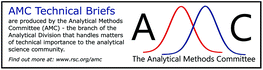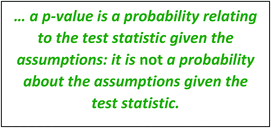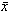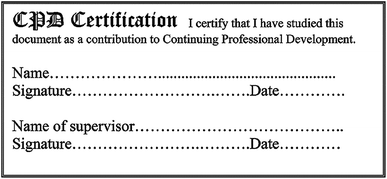# To p or not to p: the use of p-values in analytical science

Analytical Methods Committee AMCTB No. 93

First published on 31st January 2020

A significance test can be performed by calculating a test statistic, such as Student’s t or chi-squared, and comparing it with a critical value for the corresponding distribution. If the test statistic crosses the critical value threshold, the test is considered “significant”. The critical value is chosen so that there is a low probability – often 5% (for “95% confidence”) – of obtaining a significant test result by chance alone. Routine use of computers has changed this situation; software presents critical values at traditional probabilities, but now also calculates a probability, the “p-value”, for the calculated value of the test statistic. A low p-value – say, under 0.05 – can be taken as a significant result in the same way as a test statistic passing the 95% critical value. This applies to a wide variety of statistical tests, so p-values now pop-up routinely in statistical software. However, their real meaning is not as simple as it seems, and the widespread use of p-values in science has recently been challenged – even banned. What does this mean for p-values in analytical science?

## Introduction

The routine appearance of p-values has apparently led to widespread misunderstanding and misuse,1 which has even been implicated in an alleged crisis of replicability in scientific research, particularly in the medical and social sciences.2,3 Some scientific journals are discouraging the use of p-values, and one leading psychology journal has banned them outright.4 Is such a strong condemnation justified? In particular, should analytical chemists be worried about these criticisms and stop using p-values? We think not, but it is vital that users remember exactly what a p-value means and when it can be relied upon.A p-value is the probability, given certain assumptions, that a test statistic equals or exceeds its experimental value. For example, suppose an analyst is checking the calibration of an elemental analyser to see whether it is biased. She takes five readings based on a reference material and finds the difference between their mean and the reference value. She then conducts a statistical test, such as Student’s t test. Like most statistical tests, this one starts with a ‘null hypothesis’; usually that there is zero bias in this case. The software used for the test generates the ubiquitous p-value; if it is small, the analyst will usually conclude that there is evidence of bias and will take some corrective action.

This p-value is the probability of obtaining the test statistic, the calculated value of Student’s t. It is – perhaps surprisingly – not the probability that the analyser is biased. Instead, it is the probability of getting the test statistic, or a greater value, if the null hypothesis is correct and with the additional assumptions listed below. It answers the question “how probable is our value of Student’s t if the instrument really has no bias?”. Clearly, if that probability is very small, it is sensible to question the null hypothesis or some other assumption; that is why the null hypothesis is ‘rejected’ when the p-value is small. Equally, if the likelihood of our result is high we assume that there is no effect of interest, and would not usually undertake further investigations.

While this is useful, we have not addressed the exact question of interest, which is: ‘How sure are we that our instrument is unbiased, given our data?’ To answer this second question properly, we would first need to know the chances of the instrument being biased before we start the experiment, as well as the chance of getting the same result if the instrument is biased. We use hypothesis tests largely because we do not generally have this essential prior information, so must rely on a related but indirect question to decide how to proceed.## What are the usual assumptions?

Statistical tests of significance are based on several assumptions about the data. These should always be explicit, but quite often are simply taken for granted. The main assumptions are the null and alternative hypotheses. The null hypothesis (designated H0) usually asserts that there is no effect. In our example, this would usually imply that the elemental analyser’s true mean reading μx for the reference material is equal to the reference value, that is, H0: μx = xref (the experimental mean reading,, is almost certainly different from xref). The alternative hypothesis, H1, is what we accept if H0 is untenable; usually, for a bias check, H1 would simply be that the bias is not zero, i.e., that μxxref. For Student’s t, this alternative hypothesis would tell us to use critical values from a two-tailed table, because both very high and very low values signal an effect to be investigated.

The test also assumes that errors are random, independent of one another, normally distributed around zero and that the (true) standard deviation is constant within the range of interest. Other distribution assumptions would mean different probability calculations. There is also an implicit assumption that only one such test is conducted. This is important because, if we carry out the test many times, we would have a much higher chance of seeing at least one extreme value of Student’s t: we should then be very wary of interpreting p-values below 0.05 as an indication of some important finding.

## Criticisms of the p-value

We have seen that a p-value can be misinterpreted as the chance of a null hypothesis being true, but there are other cogent criticisms of the unquestioning use of p-values. These include:

– 0.05 is an arbitrary criterion, leading us to claim a ‘significant’ finding one time in 20 when there is in fact no real effect. False positive findings can lead researchers to conduct unnecessary follow-up work. Worse, in fields where the effects studied are nearly always very small or absent – for example, looking for genes with a large effect on a disease, among a very large population of genes with no effect – the outcome is that nearly all of the apparent ’positives’ (at p = 0.05 for each single experiment) will be false.

– A small, and therefore ‘significant’, p-value does not say anything about the size or practical importance of the effect; inconsequential bias can lead to a significant p-value if precision is very good.

– A small p-value is unlikely to arise by chance, but it does not rule out the possibility of experimental bias caused by some factor that is not under study. For example, runs on different stability test samples on two separate days may show a significant difference, but that need not signal a change in the test materials: it may indicate instead a change in the analytical method.

– A large p-value is not proof that there is no effect. The experiment may not be sufficiently precise, or too small, to find the effect of interest. In short, “absence of evidence is not evidence of absence”; we may simply have not looked hard enough!

– Probability calculations are based on theoretical assumptions that are almost always approximate for real data. Very small p-values rely on the ‘tails’ of a distribution, and as any proficiency test result will usually demonstrate, the tails of the data rarely follow a normal distribution closely.

p-Values can be misused very easily when seeking findings for publication. If we study one large group of data, carrying out one hypothesis test, we can stay with our familiar p < 0.05 criterion. However, if that is not met, it is easy and tempting to break the data into subsets and test all of those individually, claiming every instance of p < 0.05 as a new discovery (this, with related abuses, even has a name – “p hacking”). But repeating the test on unplanned subsets greatly multiplies the chances of individual false positives. Statisticians have long had methods of correcting for this – usually by modifying the p-value criterion to retain a low false positive rate for the experiment as a whole. Unfortunately this so-called ‘multiple testing’ correction does not feature in many science or statistics courses for analytical chemists.

## When can analytical scientists safely use p-values?

With so many concerns surrounding p-values, we might feel that we should abandon them entirely. However there are important instances, frequently arising in analytical chemistry, where they remain useful. These include:

– As an indication that follow-up action is not needed. Most criticism of p-values focus on over-interpretation of significance, but most method validations, for example, rely on insignificance; we are reassured by negative findings, not seeking positive findings. When an experiment is properly carried out, it remains safe to take an insignificant p-value as ‘no cause for concern’.

– As a signal for follow-up investigation. Again, in method validation, a significant p-value is usually followed up, both to confirm that there is a problem and because it needs to be rectified. This provides very substantial protection against inadvertent over-interpretation.

– Use in conjunction with other indicators of effect size: an insignificant p-value with a small measured effect and a small confidence interval should be reassuring evidence that an effect can genuinely be neglected.

– As a protection against visual over-interpretation. Visual inspection of data often shows apparent outliers, trends, curves or regularities because human visual systems are adept at finding anomalies. Our eyes effectively consider many possible patterns at once, and we are likely to test only for the pattern we see: this is a hidden ‘multiple testing’ problem, so a significant finding need not signal a real effect. However, with that bias towards significant findings, an insignificant hypothesis test can be a good reason to disregard the visual anomaly as chance.

Therefore for most normal validation and QC applications, the use of p-values remains justified when properly applied. The important caveat is that, if an experiment is to be used as evidence that no further work is needed, the experiment must be sufficiently powerful to find an effect that would be important (AMC Technical Brief No. 38 (ref. 5) explains test power more fully). We cannot claim that, say, three replicates with a 15% relative standard deviation are sufficient to show that there is no bias over 5%; such an experiment is simply not sufficient for that purpose. We still need 10–15 replicates to be reasonably sure of detecting a bias as large as our available standard deviation.

## Alternatives to the p-value

Many statisticians prefer to calculate confidence limits, for example based on the t-distribution, rather than just calculating p-values. A confidence interval is closely related to a significance test: our measured result would have a p-value of exactly 0.05 if tested against one extreme of a 95% confidence interval for the same hypothesis. A confidence interval improves considerably on the p-value alone by indicating a plausible range of values. This is particularly useful when the confidence interval is wide, or when the measured effect is small (and possibly unimportant) but precisely measured. However, the exact meaning of a confidence region is not easy to explain; for example, surprisingly little can be said about the chance that a given interval contains the true value.

Another useful alternative is graphical inspection. Computers provide many different graphical displays of our datasets instantly, and these may tell us (with a bit of experience) all we need to know without further ado. Significance tests are then optimally useful when visual examination seems to be marginal.

## The Bayesian dimension

Significance tests have been criticised on the grounds that we assume something as true that cannot be true exactly (the null hypothesis) to obtain something that we do not want to know anyway, the p-value being a probability relating to the experimental data. What we really would like is the probability of the null hypothesis being true, but we cannot logically derive that probability from the data alone. Bayes’ law shows that we would need additional information – a prior distribution – to do that (for Bayesian statistics see AMC Technical Briefs No.s 14 (ref. 5) and 52 (ref. 6)). In most instances in analytical chemistry, however, a straightforward frequentist significance test will suffice.

## Conclusions

• Correctly applied, p-values remain a valid method of interpreting data in most circumstances in method validation, calibration, and analytical quality control.

• An insignificant p-value is not evidence of absence of an important effect or acceptability unless the experiment is properly designed and sufficiently powerful.

• A significant p-value should be followed up and confirmed, particularly if it implies a need for expensive or safety-critical action.

• A p-value is not a probability that the null hypothesis is correct. It is the probability that the observed result would have arisen if the null hypothesis is correct.

• Statistical significance is not the only criterion on which action should be based; look at the measured effect size and the confidence interval as well.

• 0.05 may not always be a reasonable boundary for statistical significance, for instance, in forensic science.

• A p-value of 0.05 (or some other value) should not be regarded as a sharp boundary, as it is arbitrarily chosen. A value of (say) 0.07 still suggests a possible effect, hinting that a larger experiment might be valuable. A p-value of (say) 0.03 indicates statistical significance, but still with a definite probability that the null hypothesis may be true.

SLR Ellison (LGC Limited)

M Thompson (Birkbeck College, London)

This Technical Brief was prepared for the Analytical Methods Committee, with contributions from members of the AMC Statistics Expert Working Group, and approved on 23 August 2019.